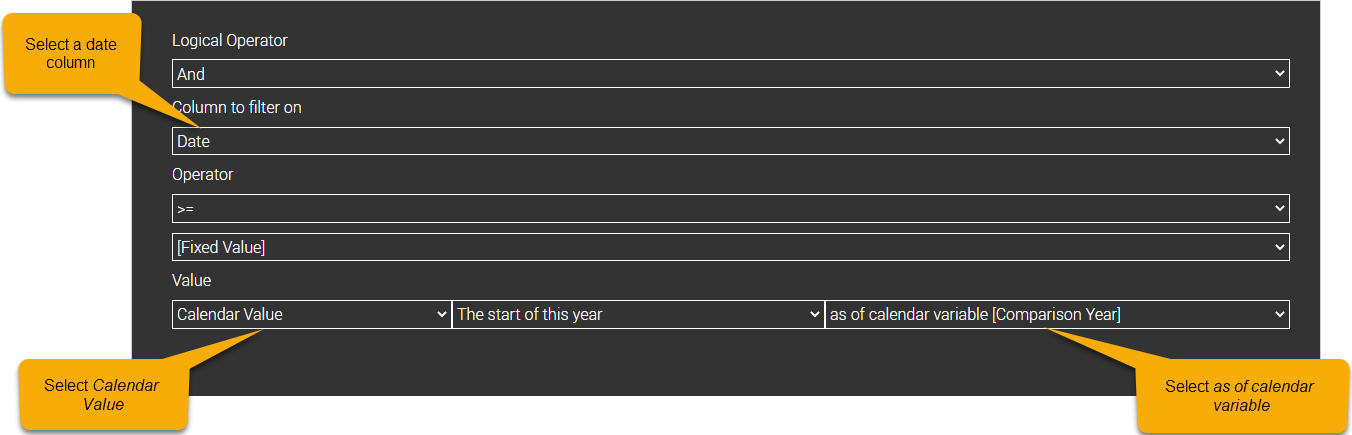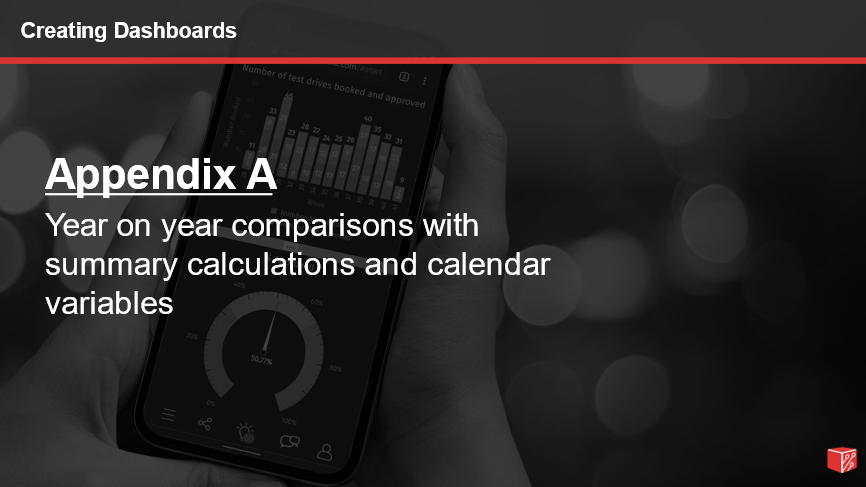## Appendix A – Year on year comparisons with summary calculations and calendar variables

### Introduction

In this lesson you will learn how to use summary calculations to easily create year on year comparisons. You will also learn how to use calendar variables to allow users to easily compare different years.

### Learning Objectives

When you complete this lesson, you will understand:

• The concept of summary calculations.
• How to create summary calculations.
• How to use summary calculations in a dashboard or visualisation.
• How to create calendar variables and apply them to filters and summary calculation.

### Prerequisites

Before you begin this lesson you should be able to:

• Access the data administration screens and edit subjects.
• Create visualisations.
• Create and open dashboards.
• Add and edit filters to dashboard objects
• Add control panels to a dashboard### Understanding summary calculations

When we add a metric to a dashboard or visualisation, the value will be calculated by applying some summary operation to each row that matches the dimensions displayed. For instance, in the chart below, the Sales Value is calculated by summing the metric Sales Value in every row that matches a particular store. A filter is applied to the chart so we only see sales for the current year.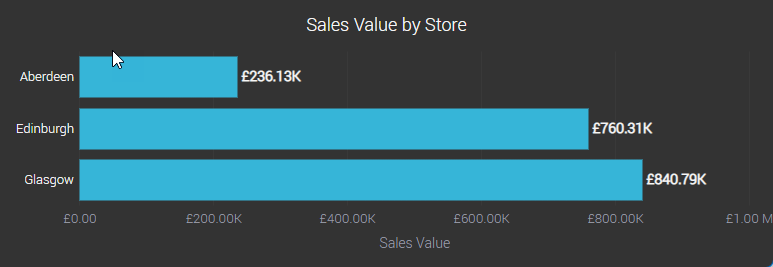However, it’s likely we might want to compare sales for each store in the current year with sales in the same stores last year. Summary calculations allow us to achieve this by creating metrics that have an implicit filter applied to them.

For instance, we could create two new metrics Sales TY, which would always only total sales in the current year, and Sales LY which would always only total sales in the previous year.

The chart below has no filters applied, however there are two implicit filters applied in the two metrics Sales TY and Sales LY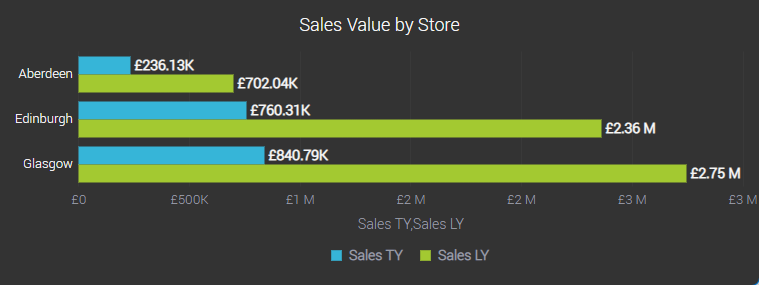### Creating summary calculations

To create a summary calculation for Sales TY, follow these steps: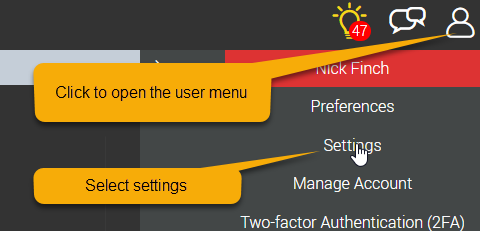2. Select Subjects in the left-hand tab, then find the subject you want to add the summary calculations to and press the edit button.3. Press the Add metric button4. Select the option Create a new metric in the subject from a new calculated column
5. Enter a name the column (we’ll use Sales LY)
6. Select Summary Calculation as the type of calculation
7. Select the column we want to sum (we’ll select Sales Value)
8. Select the summary operation (we’ll use Sum)
9. Define the filter to apply to this summary column. For our Sales LY example, we’ll do the following...
1. Press the Add Filter Condition button to add a filter condition2. Select Date as the column to filter on
3. Select >= as the operator
4. Under the Value heading, select Calendar Value
5. Select Start of this year as of 1 year ago for the calendar value options
6. Press OK7. Repeat steps a and b above to add a second condition.
Select <= as the operator
8. Under the Value heading, select Calendar Value
9. Select End of this year as of 1 year ago for the calendar value options
10. Press OK

### Using summary calculations

Once you have created a summary calculation, it’s available in visualisations and dashboards as any other metric. The implicit filter is automatically applied to any figure where the summary calculation is used.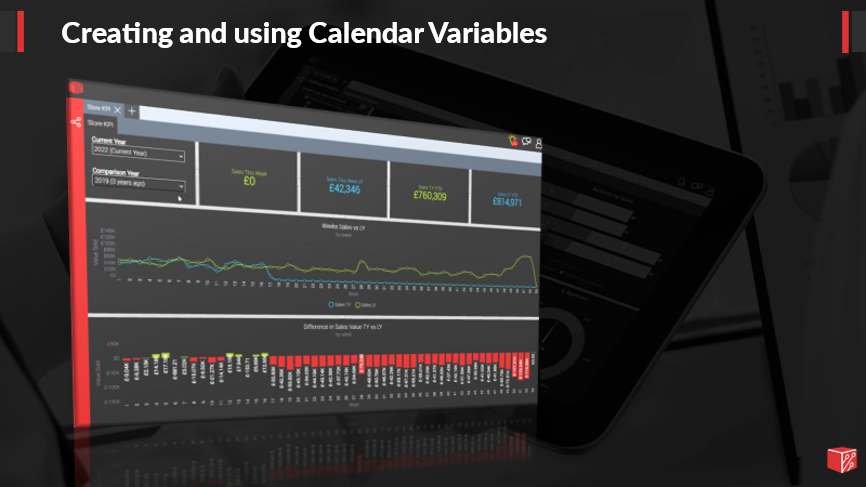### Understanding Calendar Variables

It is a common requirement to build dashboards that display year on year comparisons. Normally we will compare the current year to the previous year. However, when we have periods of unusual trading, perhaps because of a global pandemic or a structural change in the organisation, we may wish to allow the user to easily change the years that are being compared. Calendar variables allow us to display selection lists on dashboards that allow users to easily specify which calendar periods are used in filters.

### Creating Calendar Variables

To create a calendar variable, follow these steps:2. Select Subjects in the left-hand tab, then find the subject you want to add the calendar variable to and press the edit button.3. Select the Calendar Variables tab, then press the add button to add a calendar variable.4. Enter the name of the calendar variable
5. Select which financial periods you wish the user to be able to check
6. Select the default value for the calendar variable7. Press OK

### Adding Calendar Variables to a Dashboard

1. Add or edit a control panel to on a dashboard.
2. Click the Calendar Variable button to add a calendar variable to the control panel.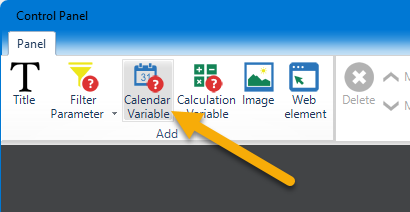3. Select the subject that contains the Calendar Variable you want to add.
4. Select the calendar that will be used to define the periods represented in the Calendar Variable
5. Select the Calendar Variable you wish to add to the dashboard.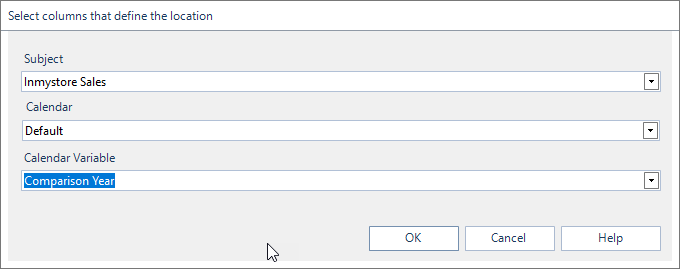6. Press OK

### Using Calendar Variables in a filter

Once a calendar variable has been added to a subject, it can be used in any filter that uses a date column in that subject. The calendar variable can then be used to change the applied filter according to the financial period selected in the calendar variable drop down.

To apply a calendar variable to a filter, do the following.

1. Edit the filter on a dashboard object.
2. Edit or add a filter condition on a date column.
3. Select Calendar Variable as the type of value
4. Select as of calendar variable [Calendar Variable Name]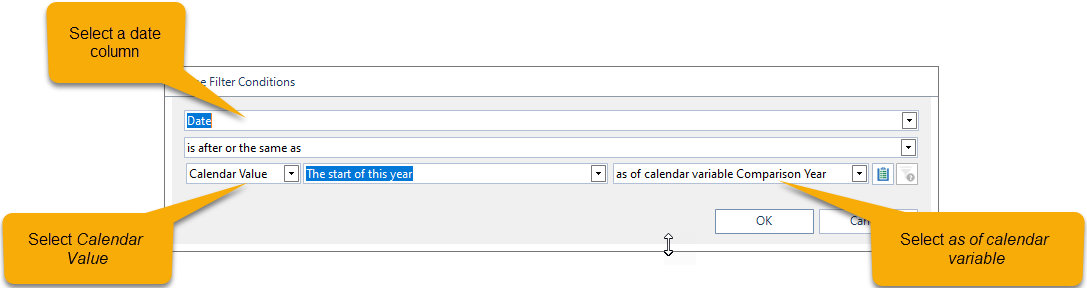### Using Calendar Variables in a Summary Calculation

To apply a calendar variable to a summary calculation, do the following.

1. Edit the filter on a summary column.
2. Edit or add a filter condition on a date column.
3. Select Calendar Variable as the type of value
4. Select as of calendar variable [Calendar Variable Name]